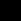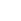## Archive for calls, October 2011[Previous message][Next message][Back to index]

# [ecrea] cfp iadis e-society conference 2012

## Wed Oct 12 09:51:41 GMT 2011

```
March 10-13, 2012 =E2=80=93 Berlin, Germany
(http://www.esociety-conf.org/)

* Conference Background and Goals
The IADIS e-Society 2012 conference aims to address the main issues of =20
concern within the Information Society. This conference covers both =20
the technical as well as the non-technical aspects of the Information =20
Society. Broad areas of interest are eSociety and Digital Divide,  =20
eBusiness / eCommerce, eLearning, New Media and E-Society, Digital =20
Services in eESociety, eGovernment /eGovernance, eHealth, Information =20
Systems,  and Information Management. These broad areas are divided =20
into more detailed areas (see below). However innovative contributes =20
that dont fit into these areas will also be considered since they =20
might be of benefit to conference attendees.

* Format of the Conference
The conference will comprise of invited talks and oral presentations. =20
The proceedings of the conference will be published in the form of a =20
book and CD-ROM with ISBN, and will be available also in the IADIS =20
Digital Library (online accessible). The best paper authors will be =20
invited to publish extended versions of their papers in the IADIS =20
Journal on WWW/Internet (ISSN: 1645-7641) and other selected Journals.

* Types of submissions
Full and Short Papers, Reflection Papers, Posters/Demonstrations, =20
Tutorials, Panels and Doctoral Consortium. All submissions are subject =20
to a blind refereeing process.

* Topics related to the Information Society are of interest. These =20
include, but are not limited to the following areas and topics:

=C2=AB=C2=AB eSociety and Digital Divide =C2=BB=C2=BB
Connectivity may imply social coherence and integration. The opposite =20
may result as well, when systematic measures are taken to exclude =20
certain individuals or certain groups. Papers are welcomed on the next =20
keywords:
=E2=80=A2 Social Integration
=E2=80=A2 Social Bookmarking
=E2=80=A2 Social Software
=E2=80=A2 E-Democracy
=E2=80=A2 Social Integration

May include issues relating to:
=E2=80=A2 Digital Goods and Services
=E2=80=A2 eCommerce Application Fields
=E2=80=A2 eCommerce Economics
=E2=80=A2 eCommerce Services
=E2=80=A2 Electronic Service Delivery
=E2=80=A2 eMarketing
=E2=80=A2 Languages for Describing Goods and Services
=E2=80=A2 Online Auctions and Technologies
=E2=80=A2 Virtual Organisations and Teleworking

=C2=AB=C2=AB eLearning =C2=BB=C2=BB
May include issues relating to:
=E2=80=A2 Collaborative Learning
=E2=80=A2 Curriculum Content Design&  Development
=E2=80=A2 Delivery Systems and Environments
=E2=80=A2 Educational Systems Design
. E-Citizenship and Inclusion
=E2=80=A2 eLearning Organisational Issues
=E2=80=A2 Evaluation and Assessment
. Political and Social Aspects
=E2=80=A2 Virtual Learning Environments and Issues
=E2=80=A2 Web-based Learning Communities

=C2=AB=C2=AB New Media and E-Society =C2=BB=C2=BB
May include issues relating to:
=E2=80=A2 Digitization, heterogeneity and convergence
=E2=80=A2 Interactivity and virtuality
=E2=80=A2 Citizenship, regulation and heterarchy
=E2=80=A2 Innovation, identity and the global village syndrome
=E2=80=A2 Internet Cultures and new interpretations of =E2=80=9CSpace=E2=80=
=9D
=E2=80=A2 Polity and the Digitally Suppressed

=C2=AB=C2=AB Digital Services in E-Society =C2=BB=C2=BB
May include issues relating to:
=E2=80=A2 Political Reporting
=E2=80=A2 Development of Digital Services
=E2=80=A2 Freedom of Expression
=E2=80=A2 E-Journalism
=E2=80=A2 Open Access

=C2=AB=C2=AB eGovernment /eGovernance =C2=BB=C2=BB
May include issues relating to:
=E2=80=A2 Accessibility
=E2=80=A2 Democracy and the Citizen
=E2=80=A2 Digital Economies
=E2=80=A2 Digital Regions
=E2=80=A2 eGovernment Management
=E2=80=A2 eProcurement
=E2=80=A2 Global Trends
=E2=80=A2 National and International Economies
=E2=80=A2 Social Inclusion

=C2=AB=C2=AB eHealth =C2=BB=C2=BB
May include issues relating to:
=E2=80=A2 Data Security Issues
=E2=80=A2 eHealth Policy and Practice
=E2=80=A2 eHealthcare Strategies and Provision
=E2=80=A2 Legal Issues
=E2=80=A2 Medical Research Ethics
=E2=80=A2 Patient Privacy and Confidentiality

=C2=AB=C2=AB Information Systems =C2=BB=C2=BB
May include issues relating to:
=E2=80=A2 Electronic Data Interchange (EDI)
=E2=80=A2 Intelligent Agents
=E2=80=A2 Intelligent Systems
=E2=80=A2 IS Security Issues
=E2=80=A2 Mobile Applications
=E2=80=A2 Multimedia Applications
=E2=80=A2 Payment Systems
=E2=80=A2 Protocols and Standards
=E2=80=A2 Software Requirements and IS Architectures
=E2=80=A2 Storage Issues
=E2=80=A2 Strategies and Tendencies
=E2=80=A2 System Architectures
=E2=80=A2 Telework Technologies
=E2=80=A2 Ubiquitous Computing
=E2=80=A2 Virtual Reality
=E2=80=A2 Wireless Communications

=C2=AB=C2=AB Information Management =C2=BB=C2=BB
May include issues relating to:
=E2=80=A2 Computer-Mediated Communication
=E2=80=A2 Content Development
=E2=80=A2 Cyber law and Intellectual Property
=E2=80=A2 Data Mining
=E2=80=A2 ePublishing and Digital Libraries
=E2=80=A2 Human Computer Interaction
=E2=80=A2 Information Search and Retrieval
=E2=80=A2 Knowledge Management
=E2=80=A2 Policy Issues
=E2=80=A2 Privacy Issues
=E2=80=A2 Social and Organizational Aspects
=E2=80=A2 Virtual Communities
=E2=80=A2 XML and Other Extensible Languages

* Important Dates:
- Submission deadline: 28 October 2011
- Notification to Authors: 28 November 2011
- Final Camera-Ready Submission and Early Registration: Until 19 December 20=
11
- Late Registration: After 19 December 2011
- Conference: Berlin, Germany, 10 to 13 March 2012

* Conference Location
The conference will be held in Berlin, Germany.

* Secretariat
Rua Sao Sebastiao da Pedreira, 100, 3
1050-209 Lisbon, Portugal
E-mail: (secretariat /at/ esociety-conf.org)/
Web site: http://www.esociety-conf.org/

* Program Committee
Program Chair
Piet Kommers, University of Twente, The Netherlands

Conference Chair
l

Committee Members:*
* for committee list please refer to =20
http://www.esociety-conf.org/committees.asp

* Co-located events
Please also check the co-located events:
Information Systems 2012 (http://www.is-conf.org/) - 10-12 March 2012
Mobile Learning 2012 (http://www.mlearning-conf.org/) - 11-13 March 2012

* Registered participants in the e-Society conference may attend =20
Information Systems and Mobile Learning conferences=E2=80=99 sessions free o=
f =20
charge.

----------------
ECREA-Mailing list
----------------
This mailing list is a free service from ECREA.
---